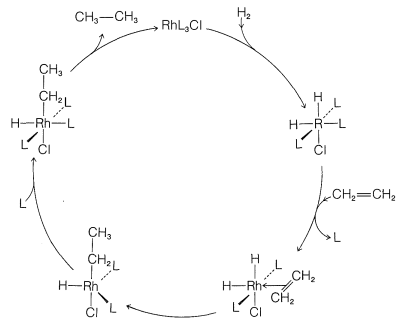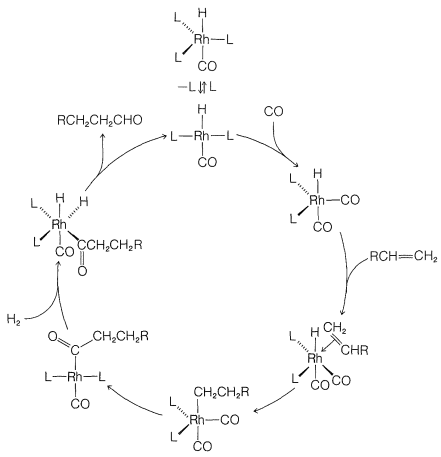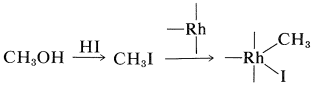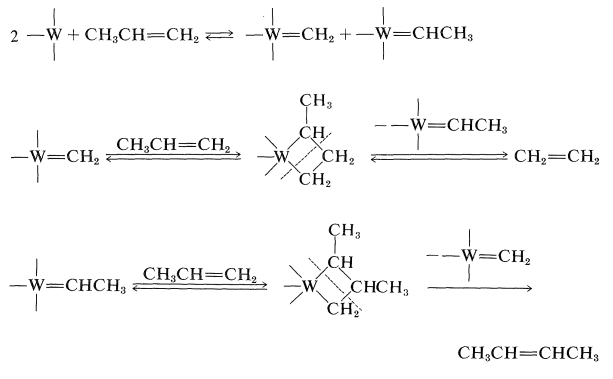# 31.4: Some Homogeneous Catalytic Reactions Involving Transition-Metal Complexes

$$\newcommand{\vecs}{\overset { \rightharpoonup} {\mathbf{#1}} }$$ $$\newcommand{\vecd}{\overset{-\!-\!\rightharpoonup}{\vphantom{a}\smash {#1}}}$$$$\newcommand{\id}{\mathrm{id}}$$ $$\newcommand{\Span}{\mathrm{span}}$$ $$\newcommand{\kernel}{\mathrm{null}\,}$$ $$\newcommand{\range}{\mathrm{range}\,}$$ $$\newcommand{\RealPart}{\mathrm{Re}}$$ $$\newcommand{\ImaginaryPart}{\mathrm{Im}}$$ $$\newcommand{\Argument}{\mathrm{Arg}}$$ $$\newcommand{\norm}{\| #1 \|}$$ $$\newcommand{\inner}{\langle #1, #2 \rangle}$$ $$\newcommand{\Span}{\mathrm{span}}$$ $$\newcommand{\id}{\mathrm{id}}$$ $$\newcommand{\Span}{\mathrm{span}}$$ $$\newcommand{\kernel}{\mathrm{null}\,}$$ $$\newcommand{\range}{\mathrm{range}\,}$$ $$\newcommand{\RealPart}{\mathrm{Re}}$$ $$\newcommand{\ImaginaryPart}{\mathrm{Im}}$$ $$\newcommand{\Argument}{\mathrm{Arg}}$$ $$\newcommand{\norm}{\| #1 \|}$$ $$\newcommand{\inner}{\langle #1, #2 \rangle}$$ $$\newcommand{\Span}{\mathrm{span}}$$$$\newcommand{\AA}{\unicode[.8,0]{x212B}}$$

## Hydrogenation

The mechanisms of hydrogenation of alkenes over finely divided metals such as nickel, platinum, and so on (Section 11-2) now are understood in a general way. However, these reactions are extremely difficult to study because they occur on a metallic surface whose structure is hard to define. In contrast, the mechanisms of hydrogenation with homogeneous catalysts are known in considerable detail and provide insight into their heterogeneous counterparts.

Homogeneous hydrogenation catalyzed by the four-coordinated rhodium complex, $$\ce{Rh[(C_6H_5)_3P]_3Cl}$$, has been particularly well investigated. With this catalyst, the first step is formation of the six-coordinated rhodium hydride of known configuration, $$16$$, in which we abbreviate the ligand, triphenylphosphine, $$\ce{(C_6H_5)_3P}$$, as $$\ce{L}$$:The next step is coordination of the alkene (here ethene) with $$16$$ with loss of $$\ce{L}$$ to give the $$\pi$$ complex, $$17$$, also of known configuration:Stable ethene complexes of $$\ce{Rh}$$ similar to $$17$$ have been isolated and shown to have the $$\pi$$-complex structure. Formation of $$17$$ must be an equilibrium process because addition of extra $$\ce{L}$$ reduces the rate of hydrogenation by shifting the equilibrium to the left.

Hydrogenation proceeds by hydride rearrangement of $$17$$ to a five-coordinated ethyl-rhodium complex, $$18$$. This complex regains a ligand molecule to replace the one lost previously, thereby giving the six-coordinated complex, $$19$$:Stable complexes with $$\ce{Rh-CH_2CH_3}$$ bonds similar to $$19$$ have been well characterized. The final step is formation of ethane from $$19$$ with regeneration of $$\ce{RhL_3Cl}$$:

$19 \rightleftharpoons \ce{RhL_3Cl} + \ce{CH_3CH_3}$

Although we abbreviate $$\ce{(C_6H_5)_3P}$$ as $$\ce{L}$$ and show little role for it or for the $$\ce{Cl}$$ attached to rhodium in the reaction, these ligands play a very important role in providing the electronic and steric environment around the rhodium, which makes efficient catalysis possible. A useful diagram of how the catalyst functions in the overall reaction is shown in Figure 31-2.Figure 31-2: Merry-go-round diagram that summarizes the steps in the hydrogenation of ethene by $$\ce{Rh[(C_6H_5)_3P]_3Cl}$$. The key features are the flow of reagents into and the products out of the cycle, the loss and regaining of the ligand, and the regeneration of the catalyst. The ligand triphenylphosphine is represented by $$\ce{L}$$.

## Hydroformylation of Alkenes (Oxo Reaction)

The conversion of alkenes to aldehydes with carbon monoxide and hydrogen in the presence of a cobalt catalyst is an important reaction (Section 16-9F):Figure 31-3: Catalytic cycle for the hydroformylation of alkenes as developed by G. Wilkinson. The stereochemical configurations of the participants in the cycle are uncertain.

## Carbonylation of Methanol

A successful commercial synthesis of ethanoic acid starts with methanol and carbon monoxide in the presence of a rhodium catalyst and hydrogen iodide:

$\ce{CH_3OH} + \ce{CO} \underset{175^\text{o}, \: 400 \: \text{psi}}{\overset{\ce{RhCl_3} \cdot 3 \ce{H_2O}, \: \ce{HI}}{\longrightarrow}} \ce{CH_3CO_2H}$

The reaction has some similarity to the hydroformylation reaction described in Section 31-4B. The hydrogen iodide is required to transform methanol to methyl iodide. The rhodium catalyst then reacts with the methyl iodide as a nucleophilic reagent:Complexation with carbon monoxide follows:A shift of the methyl group from rhodium to carbon and then hydrolysis gives the acid and regenerates $$\ce{HI}$$ and the rhodium catalyst:## The Alkene Metathesis Reaction

One of the most curious catalytic reactions of alkenes ever discovered is alkene metathesis or alkene dismutation, in which two alkenes exchange alkylidene groups, usually over a tungsten catalyst. The essence of the reaction is illustrated by a commercial process for converting excess propene to a mixture of ethene and butenes:The reaction products are those expected if cyclobutanes were intermediates, but formation and cleavage of cyclobutanes is not the correct mechanism because cyclobutanes generally are not converted to alkenes over alkene-metathesis catalysts.

After a great deal of research on the mechanism of this reaction, it now appears likely that the crucial step is the formation of carbene metal complexes and that the products are formed by recombination of the carbenes with alkene in the various possible ways: